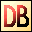DelphiBasicsDegToRadFunction Convert a degrees value to radians Math unit
 function DegToRad ( const Degrees : Extended ) : Extended;
Description
The DegToRad function is a mathematical function converting a Degrees value to Radians

PI radians = 180 degrees
Related commands
 CelsiusToFahrenheit Convert a celsius temperature into fahrenheit Convert Convert one measurement value to another FahrenheitToCelsius Convert a fahrenheit temperature into celsius RadToDeg Converts a radian value to degrees
Author links

Buy Website Traffic at
Buywebsitetrafficexperts.com

Buy Proxies at
Buyproxies.ioDownload this web site as a Windows program.

 Example code : Get the Sine of 30 degrees var   float : single; begin  // The Sine of 30 degrees = 0.5   float := Sin(DegToRad(30));   ShowMessage('Sine off 30 degrees = '+FloatToStr(float)); end; Show full unit code Sine of 30 degrees = 0.5
 Delphi Programming © Neil Moffatt 2002 - 2018. All rights reserved.  |  Home Page